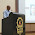## Wednesday, April 19, 2017

### P is for P-Value

Hopefully you're picking up on a recurring theme in these posts - that statistics is, by and large, about determining the likelihood that some outcome would happen by chance alone, and using that information to conclude whether something caused that outcome. If something is unlikely to occur by chance alone, we decide that it didn't occur by chance alone and the effect we saw has a systematic explanation.

We use measures like standard deviation to give us an idea of how much scores vary on their own, and we make assumptions (which we should confirm with histograms) about how the data are distributed (usually, we want them to be normally distributed). These pieces of information allow us to generate probabilities of different scores. When we conduct statistical analysis, one of the pieces of output we get is the probability that we would see the effect we saw just by chance. That, my friends, is called a p-value. We compare our p-value to the alpha we set beforehand. If our alpha is 0.05, and our p-value less than or equal to 0.05, we conclude there is a real difference/effect.

Let's use our caffeine study example once again. Say I conducted the study and found the following (note - M = mean, SD = standard deviation):

Experimental group: M = 83.2, SD = 6.1
Control group: M = 79.3, SD = 6.5

Let's also say there are 30 people in each group. This is all the information I need to conduct a simple statistical analysis, in this case a t-test, which I'll talk more about in the not-so-distant future. I conduct my t-test, and obtain a p-value of 0.02. The difference in mean test performance (between 83.2 and 79.3) has a 2% chance of happening by chance alone. That's less than 0.05, so I would conclude there is a real difference here - caffeine helped the experimental group perform better than the control group.

But 2% isn't 0. The finding could still be just a fluke, and I could have just committed a Type I error. The only way to know for certain would be to replicate the study.

1.2.